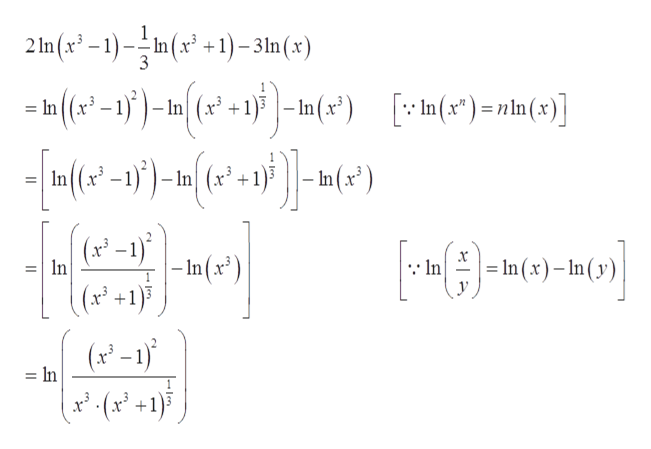# 24. 2n(ar31) In(3 +1)-3n(x)

Question
check_circleExpert Solution
Step 1

To simplify using the properties of the logarithm the expression given below:

Step 2

The properties of log function required to simplify the expression are given below:

Step 3

Simplify the expression...help_outlineImage Transcriptionclose2 In(x2-1)-In(x +1)-31n (r) In(a - j)-In| (x2 +1} | -In(x*) In (x*)=nin (x)] ()nn(x)] In ((x3 In(x - In In +1 (x-1) -In(x*) In (x)-n(y n In (x-1) = In +1 fullscreen

### Want to see the full answer?

See Solution

#### Want to see this answer and more?

Solutions are written by subject experts who are available 24/7. Questions are typically answered within 1 hour*

See Solution
*Response times may vary by subject and question
Tagged in

### Algebra